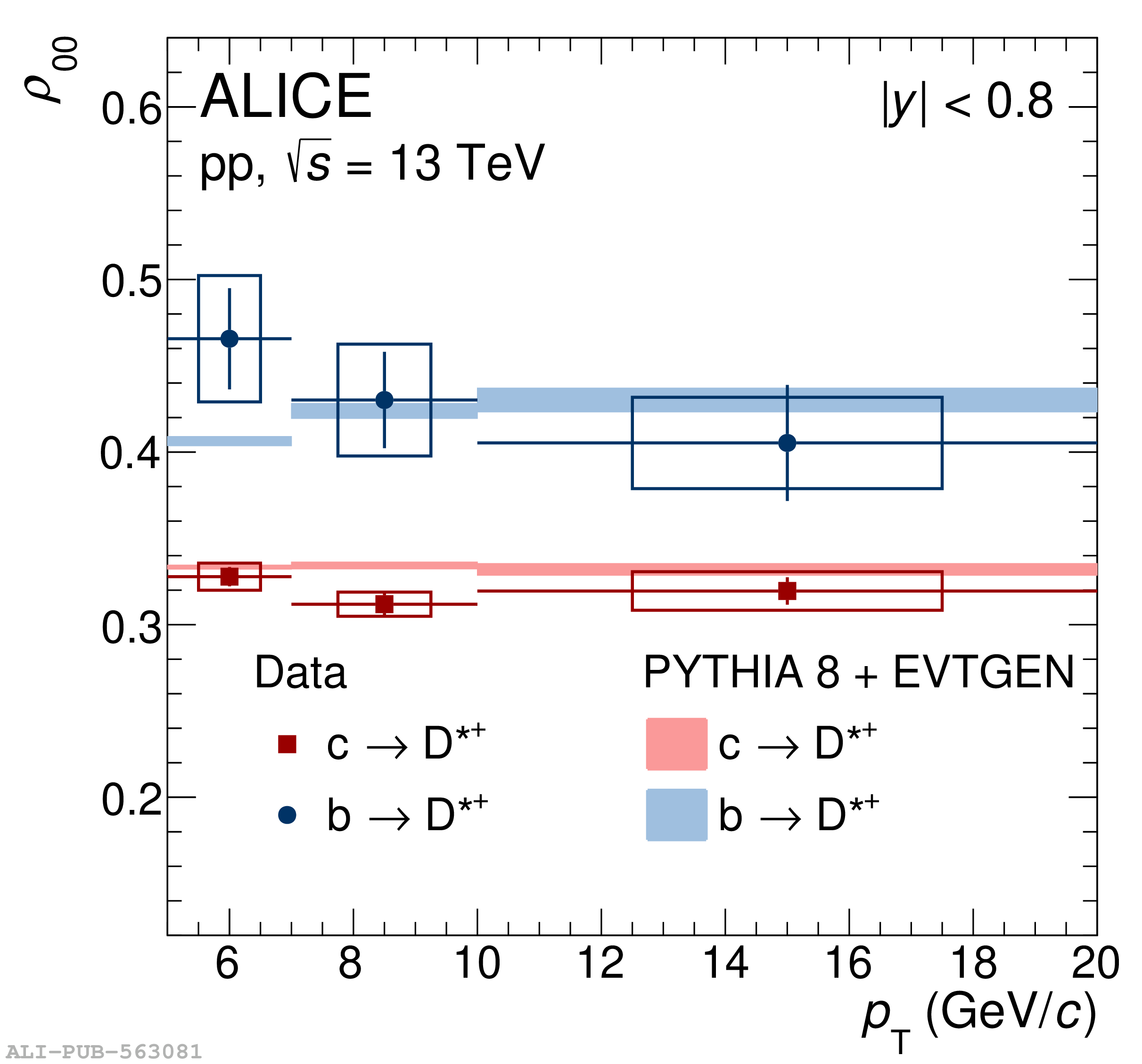# Figure 5

 The spin density matrix element (\rzz) for prompt and non-prompt \Dstar mesons as a function of \pt in pp collisions at $\s=13 \TeV$. Measurements are carried out with respect to the helicity axis at $|y|<0.8$. Statistical and systematic uncertainties are represented by bars and boxes, respectively. The measurements are compared with the estimations from the QCD-based MC event generator \pythia + \evtgen . Model estimations are shown by colour bands where the width of the band corresponds to the statistical uncertainty in model calculations.